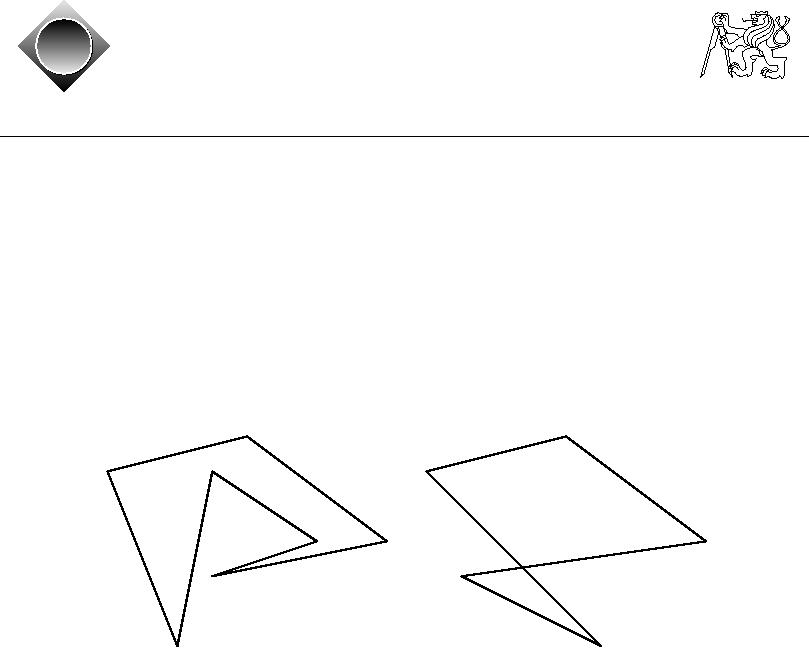Czech ACM Student Chapter
Czech Technical University in Prague
Charles University in Prague
Technical University of Ostrava
acm
ˇ a
Slovak University of Technology
Pavol Jozef Saf´rik University in Koˇice
s
cz
ˇ
University of Zilina
Masaryk University
Matej Bel University in Bansk´ Bystrica
a
University of West Bohemia
CTU Open Contest 2011
Simple Polygon
polygon.c, polygon.C, polygon.java, polygon.p
A polygon P determined by points p1, p2, . . . , pn is a closed chain of line segments (called
edges) p1p2, p2p3, . . . , pnp1 in the plane. Polygon P is simple, if no two edges have any points
in common, with the obvious exception of two consecutive segments having one common point
(called vertex). Note however, that if a vertex is part of any other (third) edge, the polygon is
no longer simple.
Any polygon that is not simple is called self-intersecting. In two example figures below, the first
polygon is simple, the second one is self-intersecting.
Your task is to determine whether a given polygon is simple or self-intersecting.
Input Specification
The input contains several test cases. Each test case corresponds to one polygon. First line of
the test case contains N , the number of points (1 N 40 000). Each of the following N lines
contains coordinates of point Pi, that is Xi, Yi separated by space, 1 Xi, Yi 30 000.
The last test case is followed by a line containing zero.
Output Specification
For each test case output either "YES" (the polygon is simple) or "NO" (the polygon is self-
intersecting).
Sample Input
5
1
6
5
7
9
4
2
3
6
1
7
1
6
5
7
9
4
4
3
7
4
4
6
3
1
7
1
1
1
4
1
3
2
2
3
1
3
3
2
2
0
Output for Sample Input
NO
YES
NO